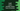# How to return multiple values from a function in Swift## How to return multiple values from a function in Swift:

We can return multiple values from a swift function. It can be achieved by returning a tuple. Using a tuple, we can group multiple values of different types and we can return that. Each element can be accessed by its name.

### Example to return multiple values from a function:

Let’s take a look at the below program:

``````func getUserInfo() -> (name: String,age: Int) {
return ("Alex", 20)
}

let user = getUserInfo()

print(user.name)
print(user.age)``````

Here,

• We are returning one string and one integer value from the function getUserInfo
• We are printing the values by accessing them by their names.

We can also return values from a function without using name:

``````func getUserInfo() -> (String, Int) {
return ("Alex", 20)
}

let user = getUserInfo()

print(user.0)
print(user.1)``````

It will print the below output for both of the above programs:

``````Alex
20``````

### Returning optional values:

We can return optional values from a function. We can have multiple optionals returning from a function. For example:

``````func getUserInfo() -> (name: String?, age: Int?) {
return ("Alex", nil)
}

let user = getUserInfo()

print(user.name)
print(user.age)``````

It will print:

``````Optional("Alex")
nil``````

Even if we mark return values as optional, we need to return values from a function.

### Returning optional tuple:

Instead of returning optional values from a tuple, we can mark the whole tuple as optional as like below :

``````func getUserInfo() -> (name: String, age: Int)? {
return nil
}

let user = getUserInfo()

print(user)``````

For this example, we can return the tuple or nil.